# DC Bridge Circuits

## DC Electric Circuits

• #### Question 1

 Don’t just sit there! Build something!!

Learning to mathematically analyze circuits requires much study and practice. Typically, students practice by working through lots of sample problems and checking their answers against those provided by the textbook or the instructor. While this is good, there is a much better way.

You will learn much more by actually building and analyzing real circuits, letting your test equipment provide the “answers” instead of a book or another person. For successful circuit-building exercises, follow these steps:

1. Carefully measure and record all component values prior to circuit construction.
2. Draw the schematic diagram for the circuit to be analyzed.
3. Carefully build this circuit on a breadboard or other convenient medium.
4. Check the accuracy of the circuit’s construction, following each wire to each connection point, and verifying these elements one-by-one on the diagram.
5. Mathematically analyze the circuit, solving for all values of voltage, current, etc.
6. Carefully measure those quantities, to verify the accuracy of your analysis.
7. If there are any substantial errors (greater than a few percent), carefully check your circuit’s construction against the diagram, then carefully re-calculate the values and re-measure.

Avoid very high and very low resistor values, to avoid measurement errors caused by meter “loading”. I recommend resistors between 1 kΩ and 100 kΩ, unless, of course, the purpose of the circuit is to illustrate the effects of meter loading!

One way you can save time and reduce the possibility of error is to begin with a very simple circuit and incrementally add components to increase its complexity after each analysis, rather than building a whole new circuit for each practice problem. Another time-saving technique is to re-use the same components in a variety of different circuit configurations. This way, you won’t have to measure any component’s value more than once.

• #### Question 2

Calculate the output voltages of these two voltage divider circuits (VA and VB):Now, calculate the voltage between points A (red lead) and B (black lead) (VAB).

• #### Question 3

Calculate the output voltages of these two voltage divider circuits (from point A to ground, and from point B to ground:Now, calculate the voltage between points A (red lead) and B (black lead).

• #### Question 4

How much voltage needs to be dropped across resistor R1 in order to make voltage VAB equal to zero?How much resistance must R1 possess in order to drop that amount of voltage?

• #### Question 5

A thermistor is a special resistor that dramatically changes resistance with changes in temperature. Consider the circuit shown below, with two identical thermistors:The “ to” label in each one shows that they both have positive α coefficients.

How much voltage would you expect the voltmeter to register when the two thermistors are at the exact same temperature? Which thermistor would have to become hotter in order to cause the voltmeter to read a significant negative voltage?

• #### Question 6

In general terms, describe what must be done to balance this bridge circuit. What, exactly, does the term “balance” mean in this context?Also, write an equation containing only the four resistor values (R1, R2, R3, and R4) showing their relationship to one another in a balanced condition.

• #### Question 7

Identify the most important qualification for the “null” meter used to balance a bridge circuit. In other words, describe what type of meter we would be looking for if we were to select one for use as a “null” meter. Describe why this particular quality is important.

• #### Question 8

What will happen to the voltage between points A and B if the power supply voltage increases?• #### Question 9

Explain how this bridge circuit is capable of being “balanced” for any values of R1 and R2:• #### Question 10

Complete the wire connections necessary to make this a bridge circuit, where [(R1)/(R2)] = [(R3)/(R4)] at balance: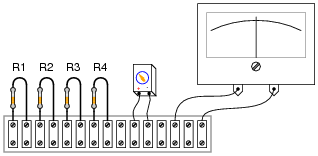• #### Question 11

In the early days of electrical metrology, the best way to measure the value of an unknown resistance was to use a bridge circuit. Explain how a four-resistor bridge (a “Wheatstone” bridge) could be used to accurately measure an unknown resistance. What components would this bridge circuit have to be constructed from? Did the power source have to be precision as well? Did the voltmeter in the middle of the bridge have to be accurately calibrated?

• #### Question 12

A strain gauge is a device used to measure the strain (compression or expansion) of a solid object by producing a resistance change proportional to the amount of strain. As the gauge is strained, its electrical resistance alters slightly due to changes in wire cross-section and length.

The following strain gauge is shown connected in a “quarter-bridge” circuit (meaning only one-quarter of the bridge actively senses strain, while the other three-quarters of the bridge are fixed in resistance):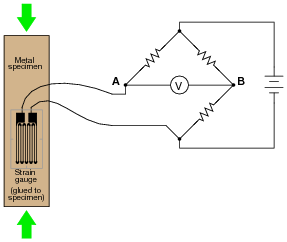Explain what would happen to the voltage measured across this bridge circuit (VAB) if the strain gauge were to be compressed, assuming that the bridge begins in a balanced condition with no strain on the gauge.

• #### Question 13

A strain gauge is a device used to measure the strain (compression or expansion) of a solid object by producing a resistance change proportional to the amount of strain: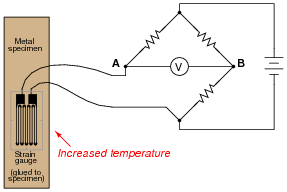The bridge circuit is supposed to respond to changes in specimen strain, but explain what will happen to the voltage measured across this bridge circuit (VAB) if the specimen’s temperature increases (with no stress applied), assuming that the bridge begins in a balanced condition with no strain on the gauge, at room temperature. Assume a positive α value for the strain gauge conductors.

What does this indicate about the effectiveness of this device as a strain-measuring instrument?

• #### Question 14

Predict how the operation of this thermistor bridge circuit will be affected as a result of the following faults. Consider each fault independently (i.e. one at a time, no multiple faults):Thermistor R1 fails open:
Thermistor R3 fails open:
Solder bridge (short) across thermistor R3:
Resistor R2 fails open:
Resistor R4 fails open:

For each of these conditions, explain why the resulting effects will occur.

• #### Question 15

Predict how the voltage polarity between test points A and B will be affected as a result of the following faults. Consider each fault independently (i.e. one at a time, no multiple faults):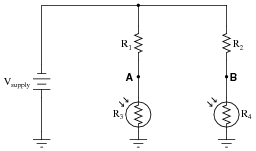Photoresistor R4 fails open:
Photoresistor R3 fails open:
Solder bridge (short) across photoresistor R4:
Resistor R2 fails open:
Resistor R1 fails open:

For each of these conditions, explain why the resulting effects will occur.

• #### Question 16

This bridge circuit is suppose to generate an output voltage proportional to the difference between light exposure on the two photocells:However something has failed in this circuit, because the voltmeter is “pegged” fully negative and will not change with varying light exposures on the two cells. Identify at least two possible failures that could cause the voltmeter to over-range in the negative direction.

• #### Question 17

Explain how this strain gauge circuit exploits a property of bridge circuits to provide automatic temperature compensation (so that changes in specimen temperature do not compromise strain measurement accuracy):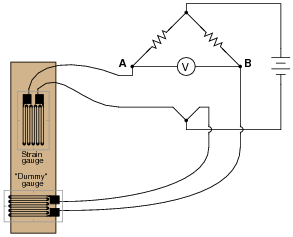• #### Question 18

The following bridge circuit uses two strain gauges (one to measure strain, the other to compensate for temperature changes), the amount of strain indicated by the voltmeter in the center of the bridge. Unfortunately, though, it has a problem. Instead of registering a very small voltage as it normally does, the voltmeter shows a large voltage difference, with point A positive and point B negative: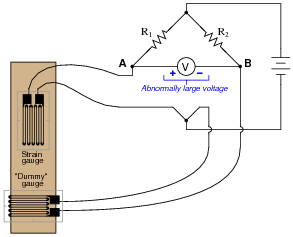Something is wrong in the bridge circuit, because this voltage is present even when there is no physical stress on the specimen. Identify which of the following faults could cause the excessive voltage to appear across the voltmeter, and which could not. Consider only one of these faults at a time (no multiple, simultaneous faults):

Resistor R1 failed open
Resistor R1 failed shorted
Resistor R2 failed open
Resistor R2 failed shorted
Strain gauge (measurement) failed open
Strain gauge (measurement) failed shorted
“Dummy” gauge (temperature compensation) failed open
“Dummy” gauge (temperature compensation) failed shorted
Voltage source is dead (no voltage output at all)
• #### Question 19

The following bridge circuit uses two strain gauges (one to measure strain, the other to compensate for temperature changes), the amount of strain indicated by the voltmeter in the center of the bridge. Unfortunately, though, it has a problem. Instead of registering a very small voltage as it normally does, the voltmeter shows a large voltage difference, with point B positive and point A negative: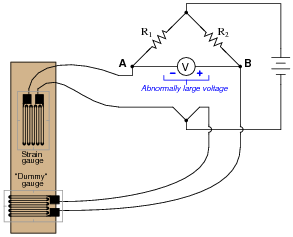Something is wrong in the bridge circuit, because this voltage is present even when there is no physical stress on the specimen. Identify which of the following faults could cause the excessive voltage to appear across the voltmeter, and which could not. Consider only one of these faults at a time (no multiple, simultaneous faults):

Resistor R1 failed open
Resistor R1 failed shorted
Resistor R2 failed open
Resistor R2 failed shorted
Strain gauge (measurement) failed open
Strain gauge (measurement) failed shorted
“Dummy” gauge (temperature compensation) failed open
“Dummy” gauge (temperature compensation) failed shorted
Voltage source is dead (no voltage output at all)